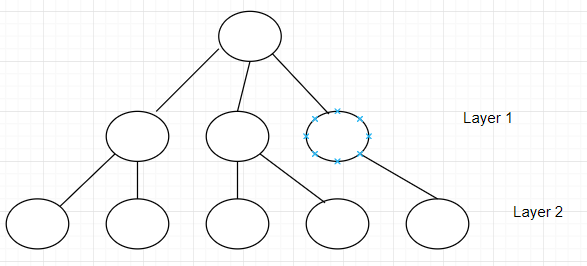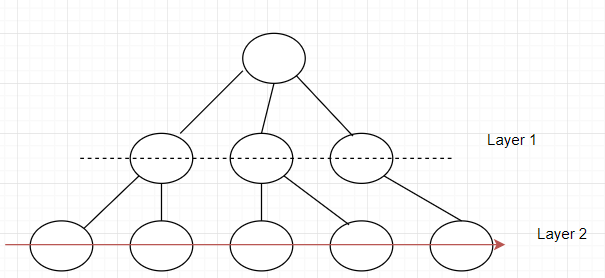Searching or traversing is really important when it comes to accessing data from a given data structure. There are different methods of traversing/searching elements within these data structures such as Graphs and Trees.Breadth-first search is one example of these methods. BFS is an algorithm that traverses tree or graph and it starts from the tree root (the topmost node in a tree) or simply the top, and scans through all of the neighbour nodes at the current depth before moving on to the nodes/elements at the next depth level.

Simply put, BFS has to complete a layer, and move on to the next until there are no any layers left.

BFS uses the exact opposite workflow as depth-first-search, which goes the other way around.

A big difference in terms of the implementation between BFS and DFS is that BFS uses queues and DFS uses stacks.

## Implementation of BFS

There are two simple rules to follow when implementing BFS:

1. Visit every single element on a given layer
2. Move on to the next layer

An example:Before moving on to layer 2, layer 1 has to be gone through first.Pseudocode

```public void breadthFirstSearch(Graph G, Object S)
// G => Graph ; S => Source node
{
define a queue named as Queue;
insert the source node in the Q
mark s as visited

perform a while loop that keeps looping until the queue is not empty
removing the current element from the queue as it will be visited now

perform a for loop that goes through all neighbours of the current element
if condition that checks if the current element/node/vertex is not visited
if it has not been visited, enqueue it and mark it as visited
}```

Actual code implementation

```public void BFS(int s, int l)
{
// create an array that holds boolean values that will have length 'l'
boolean visited[] = new boolean[l];

// create a queue

// mark the current node as visited and add it to the queue
visited[s]=true;

while (q.size() != 0)
{
// dequeuing a vertex from queue
s = q.poll();

// get all adjacent vertices of the dequeued vertex  if a adjacent has not
// been visited, then mark it visited and enqueue it
while (k.hasNext())
{
int j = k.next();
if (!visited[j])
{
visited[j] = true;